Next: 5.2.2 Cylindrical potential Up: 5.2 Pattern association algorithm Previous: 5.2 Pattern association algorithm

## 5.2.1 Spherical potential

First, we consider a spherical potential field in feature space. Here, it is not necessary to compute the principal components. All we need is the mean of the data in the feature space,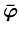=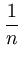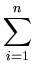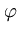(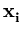) . (5.1)

The potential of a point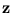in the original space is the squared distance from its mapping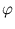() to the center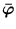,

 ES(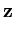) = ||() -||2 =()T() - 2()T+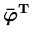. (5.2)

Using (5.1), the scalar products can be replaced by the kernel function k (see section 2.4),

 ES() = k(,) -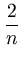k(,) +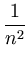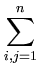k(,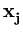) . (5.3)

All parts of this equation are known. The last term is constant, and can therefore be omitted. For radial basis functions (section 2.4.3), the first term is also constant, and the potential can be simplified to:

 ES() = -k(,) . (5.4)

This function is proportional to the Parzen window density estimator (Parzen, 1962).Next: 5.2.2 Cylindrical potential Up: 5.2 Pattern association algorithm Previous: 5.2 Pattern association algorithm
Heiko Hoffmann
2005-03-22